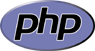You are here:

# Beginner’s Guide to PHP – Part Two

|  PHP

Let’s have a quick re-cap of what we covered in Beginner’s Guide to PHP – Part One: there are different data types (string, integer, floating point number and boolean) and variables are created by simply assigning data to them. Simple enough? Well, now we’re going to take a look at mathematical operators and what we can do with them…

### Mathematical Operators

‘Operator’ is the term used to cover various fancy symbols that we use to do stuff in PHP. (Nicely vague, right?) That is, we can use them to add integers, compare variable values, etc.. Easiest to understand are the mathematical operators — these are the kind you’re used to seeing on a calculator — and are as follows:

 + Used to add two integers (numbers) together – Used to subtract a number from another * Used to multiply two numbers together / Used to divide a number from another % Used to find the remainder after two numbers are divided from each other

At the end of Part One we also assigned data to two variables, like so:

```<?php
\$name = "Jem";  // this is a string
\$age = 20;  // this is an integer
?>
```

(Those of you who are really clever will have also noticed that I demonstrated a type of PHP comment without explaining what they were, but let’s not pick on the inconsistencies… I’ll cover PHP comments later on, when I figure out where to incorporate them. Anyway…)

We can use the \$age variable we created to test our mathematical operators. Firstly, ‘+’, because I’m not 20 anymore, so we’ll want to add a year (how convenient). There are two ways to do this, and which method you choose depends on whether or not you want to retain the original value. For example, if you want to keep \$age as 20 and want a new variable to store our new age, we can do this like so:

```<?php
\$name = "Jem";  // this is a string
\$age = 20;  // this is an integer
\$newAge = \$age + 1;  // this is our age (20), plus 1 year
?>
```

Here we are adding 1 to our original variable. Of course, we don’t need a new variable, so we might as well just add to straight to our \$age variable:

```<?php
\$name = "Jem";  // this is a string
\$age = 20 + 1;  // this is an integer
?>
```

In reality, we all know that 20 + 1 is 21 and getting PHP to do this mundane task is a bit boring. However, it’s good to understand that it’s possible to do your maths homework with a script. The other mathematical operators work in the same way: `\$age = 20 * 5;` would assign the value of 20 * 5 (which is 100) to our \$age variable and so on.

Once you’ve created your variables and done some maths magic, you might want to display those variables to the browser. This is where the `echo` statement comes in handy. (There is also `print`, but the `echo` vs. `print` debate is long, and I use `echo` out of habit.)

### `echo` Statement

`echo` is what’s known as a simple statement. We use it to output everything inside the single or double quotation marks (…getting to that bit) or using the “here document” syntax. The contents an `echo` statement can span multiple lines and use infinite amounts of whitespace. BUT, the statement needs to end with a semi-colon (much like assigning variables) otherwise you get a parse error.

As I said, the contents that we want to output needs to be inside quotation marks. What you use depends on the affect you want. For example, double quotation marks will output everything — and — if there’s a variable in the string you’re trying to `echo` it will show the value of the variable (e.g. `<?php echo "Hello \$name"; ?>` will output Hello Jem). However, if you use single quotation marks, you must ‘break out’ to `echo` a variable, otherwise all you will see is the variable name (e.g. `<?php echo 'Hello \$name'; ?>` will output Hello \$name).

Alternative to the quotation marks is the aforementioned “here document” syntax. As the PHP manual has a very good block of `echo` examples, I’m not going to repeat the information unnecessarily — see example 2298, echo().

Now that we’ve come to grips with `echo` and mathematical operators we can use the two to create a string of text, and output it to our browser.

```<?php
\$name = "Jem";  // this is a string
\$age = 20 + 1;  // this is an integer
echo "My name is \$name and my new age is \$age";
?>
```

Assuming you’ve copied that into a .php file and your server supports PHP as we discussed in Part One, you should see My name is Jem and my new age is 21. Magic — our first little script that outputs data to the browser.

In Part Three I will cover the other types of Operators and how they can be used (and hopefully not take 6 months to do it!)This article is part of the Beginner’s Guide to PHP series. You can see all the guides or jump to Part Three.
Jem Turner jem@jemjabella.co.uk +44(0)7521056376